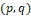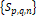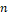### Generalized (p,q)-Fibonacci-Like Sequences and Their Properties

•  Yasemin Taşyurdu

#### Abstract

In this paper, we define the generalized (p,q)-Fibonacci-Like sequences Sp,q,nassociated with the (p,q)-Fibonacci and the (p,q)-Lucas sequences and then we get some fundamental identities and sums formulas involving odd and even terms of the generalized (p,q)-Fibonacci-Like sequences. We obtain the Binet's formula to find the nth general term of generalized (p,q)-Fibonacci-Like sequences Sp,q,n. Also, the generating functions of these sequences are presented and proved.In this paper, we define the generalized (p,q)-Fibonacci-Like sequences Sp,q,nassociated with the (p,q)-Fibonacci and the (p,q)-Lucas sequences and then we get some fundamental identities and sums formulas involving odd and even terms of the generalized (p,q)-Fibonacci-Like sequences. We obtain the Binet's formula to find the nth general term of generalized (p,q)-Fibonacci-Like sequences Sp,q,n. Also, the generating functions of these sequences are presented and proved.This work is licensed under a Creative Commons Attribution 4.0 License.
• ISSN(Print): 1916-9795
• ISSN(Online): 1916-9809
• Started: 2009
• Frequency: bimonthly

### Journal Metrics

• h-index (February 2019): 18
• i10-index (February 2019): 48
• h5-index (February 2019): 7
• h5-median (February 2019): 10